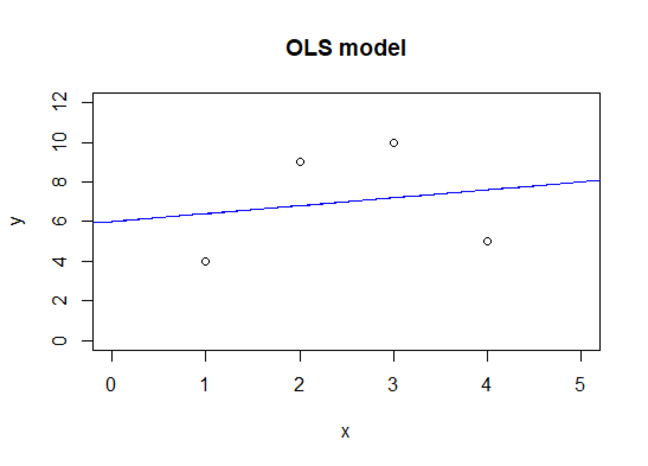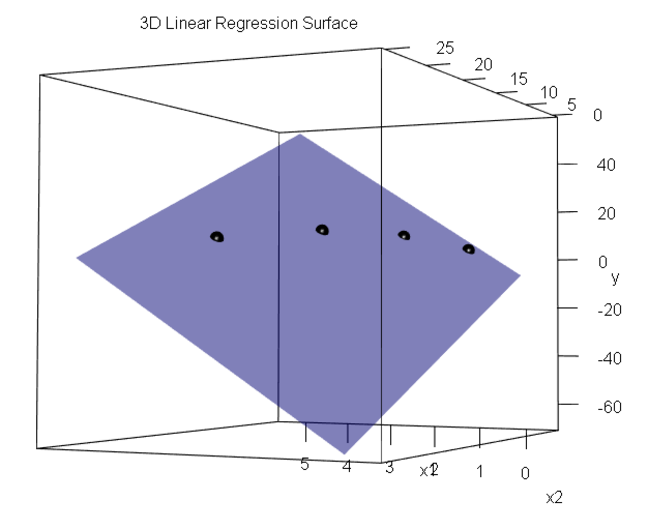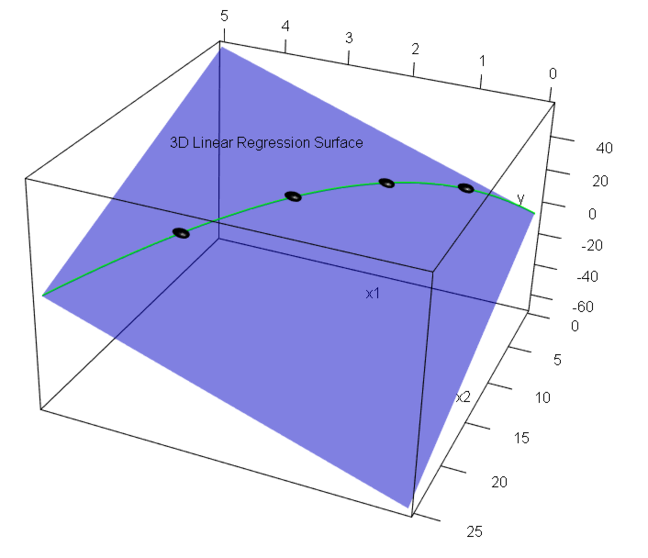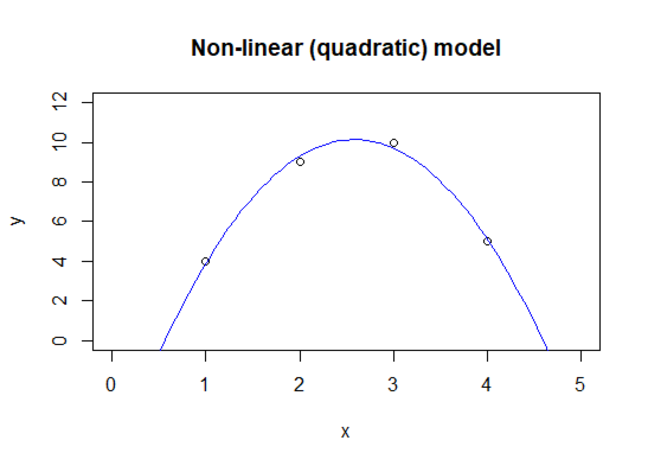## Want to keep learning?

This content is taken from the The Open University & Persontyle's online course, Advanced Machine Learning. Join the course to learn more.
1.9

# Linear Models, Non-Linear Models & Feature Transformations

## Linear Models

A linear model is one that outputs a weighted sum of the inputs, plus a bias (intercept) term. Where there is a single input feature, X, and a single target variable, Y, this is of the form:

$f(X) = \beta_0 + \beta_1 X$

This 2-dimensional case generalizes to n variables.

$f(X) = \beta_0 + \beta_1 X_1 + \beta_2 X_2 + ... + \beta_n X_n$

Notice that the intercept, $$\beta_0$$ is unusual for the parameters of the model in that it is not a coefficient of any $$X$$ variable. To allow for simpler representation, we can introduce a ‘dummy’ variable $$X_0$$ which always takes the value of 1. We now treat $$\beta_0$$ as the coefficient of $$\X_0$$. Note that whenever in this course you see $$X_0$$, i.e. an $$X$$ variable with a zero index, this will be taken to be a dummy variable with constant value 1.

This allows us to represent the previous formula as:

$f(X) = \sum_{i=0}^n \beta_i X_i$

Or, in matrix notation:

$f(X) = X \beta$

Graphically, a linear model produces:

• a point in 1-dimension (no features)
• a line in 2-dimensions (one feature)
• a plane in 3-dimensions (two features)
• a hyperplane in n-dimensions (n-1 features)

It is clear how such a model functions as a regression model - the output is simply the estimate of the value for the target variable and the hyperplane is the regression curve. They can also be binary classifiers. In such linear classifiers, the hyperplane given by the model specifies a decision boundary rather than a regression curve.

Regression case: $$\hat Y = f(X)$$

Classification case: $$\hat Y = 1, if f(X) > 0\\$$ $$0, otherwise$$

## Non-Linear Models

Linear models have a number of advantages: They are easy to interpret, and fast to train and use, since the mathematics involved is simple to compute. Unfortunately, though, the real world is seldom linear. This means that linear models are normally too simple to be able to adequately model real world systems. Instead, we often need to use non-linear models.

## Non-Linear Transformations

Let us assume we have the data given below. We wish to generate a model that estimates the value of Y given X.

X Y
1 4
2 9
3 10
4 5

Using OLS we generate the following linear model:

$\hat Y = 6 + .4 X$

With the regression curve:However, instead of looking for a linear relationship between X and Y, we could look for a linear relationship between the transformations of X and Y. This is entirely legitimate. By transformation we simply mean functions of X, and any function of a random variable (or set of random variables) is itself a random variable. (Although we only have one input feature in this example, note that in the general case such each transformation function would be arbitrary functions of all input features.)

Let us consider two such transformation functions of X:

$f_1(X)=X$ $f_2(X)=X^2$

Applying these in our case gives us the new data:

F_1=X F_2=X^2 Y
1 1 4
2 4 9
3 9 10
4 16 5

The $$F_1$$ and $$F_2$$ columns of this new data set are latent variables (a function of a random variable, or set of random variables, is itself a random variable). We can now consider the relationship between our target variable and these latent variables. Using OLS to model this relationship we generate the following model:

$\hat Y = -6.5 + 12.9 X - 2.5 X^2$

Now, the regression plane in 3-dimensions is:This is, of course, a linear surface in 3-dimensions: $$X, X^2 and Y$$. But consider that most of the points on this plane are impossible, since they correspond to values x on the X axis and z on the $X^2$ axis such that $$x^2 \neq z$$. In fact, there is only a single curve of valid points: Those where $$x^2 = z$$. This curve is non-linear, and if we pick it out:We can plot it in the data space corresponding to our original data:We have thereby obtained a non-linear model in our original data by combining a linear method with non-linear transformation of our original data. This approach is one that is we will encounter repeatedly being used to turn both linear regression and linear classification models in much more flexible non-linear models. The key to understanding what is going on is that we are producing a linear model in a high dimensional space where the data coordinates are given by non-linear transforms of the original input features. This results in a linear surface in the higher dimensional space. But when we look for legitimate values of the coordinates in this data space, we obtain a non-linear ‘hypercurve’ along that linear surface. This ‘hypercurve’ is non-linear precisely because our transformations were non-linear.